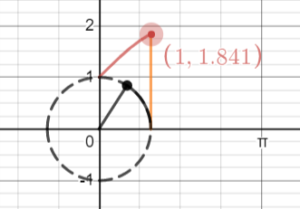# Covercosine Function (Coversed Cosine)

Contents:

## Covercosine

The covercosine function (coversed cosine) is a periodic function, written as covercosine(θ) , covercos(θ) or cvc(θ). It has the formula:

covercosine(θ) = 1 + sin(θ).

## Covercosine in Visual Terms

The covercosine is shown on the following unit circle (a circle with a radius of 1), for θ = 1:I created this graph with Desmo.com’s “Unraveling Trig Functions” calculator, which allows you to change values like the arc lengths. The calculator gives a host of great visuals to show how the covercosine compares to more familiar functions, like the sine function and cosine function. It’s well worth a look if you’re a visual learner.

Another way to look at the covercosine is as a shifted function. As an example, the more familiar cosine function can be thought of as a shifted sine function, as the following image illustrates:

Of course, you’d have to be familiar with the vercosine to fully understand what it means to shift it, but hopefully you get the idea: dozens of trigonometric functions were once in use that could measure any angle and any distance for segments of a trip. They were invaluable to seafarers, who could calculate how far and in what direction, based on the simple idea of breaking apart a circle into smaller components.

## History of the Covercosine Function

Covercosine is one of a group of archaic functions (along with hacoversine, vercosine and others) used historically for navigation. With the advent of modern navigation techniques, these functions have fallen out of practical use along with the typewriter, abacus, and slide rule. Thanks to computing, most trigonometric functions still in use are derived from sine functions and cosine functions or from complex exponential functions.

## Hacovercosine

Hacovercosine (also called cohavercosine or hacoversed cosine and abbreviated to hcc) is short for halved-coversed-cosine. It’s literally half the covercosine function.

The formula is
1 + sin(θ)/2
or, more simply, half the covercosine:
coverscosine(θ)/2.

It is a type of sine wave that oscillates between 0 and 1.## History of the Hacovercosine

This archaic trig function was primarily used for global navigation, back in the days when bulging books of navigational tables were a must-have on ships. They can also can be found in older text books. If your calculations involved finding half of the square of cosine, you could look that up in a table and not have to deal with finding halves of squares and square roots. Nowadays, this function is of little practical use, thanks to modern computing. However, it does pop up now and again in the scholarly literature, like in 2013’s On the Trigonometric Loophole or 2019’s Computational Aspects of q-Method.

## References

Sine wave image adapted from: Cmglee|Wikimedia Commons. CC BY-SA 4.0.
What in the world is a hacovercosine? Retrieved December 6, 2019 from: http://www2.hawaii.edu/~tvs/trig.html
Desmos Graphing Calculator.

CITE THIS AS:
Stephanie Glen. "Covercosine Function (Coversed Cosine)" From StatisticsHowTo.com: Elementary Statistics for the rest of us! https://www.statisticshowto.com/covercosine-function/